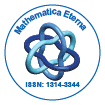# Mathematica EternaOpen Access

ISSN: 1314-3344

## Abstract

### The Fractional Schr¨odinger-Klein-Gordon Equation and Intermediate Relativism

Jonathan Blackledge and Bazar Babajanov

By considering a random walk model compounded in Einstein’s evolution equation, we show that both the classical Schr¨odinger and Klein-Gordon equations can be viewed as a consequence of introducing a memory function given by −iδ and δ (1), respectively. For a memory function of the type −i 1+αδ (α) where 0 < α < 1 we derive a fractional Schr¨odinger-Klein-Gordon equation whose corresponding propagator (free space Green’s function) is then evaluated. The purpose of this is to derive a wave equation that, on a phenomenological basis at least, describes the transitional characteristics of wave functions for spin-less particles that may exist in the intermediate or ‘semirelativistic’ regime. On the basis of the phenomenology considered, it is shown that such wave functions are self-affine functions of time t with a probability density that scales as 1/t1−α for mass-less particles.

Top